Lehigh University / AT&T High School Math Contest
March 27, 1999

1. Do not open this exam until you are told to do so.
2. Print your name clearly on your answer sheet. On the "Grade" line, write
your grade in school (9,10,11, or 12) not the grade level of your team.
3. Print clearly on the answer sheet your answer to each question. You should
not write decimal approximations to numbers such as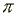,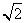and 10!.
You should simplify your answers as much as seems reasonable. For example
6/4 should be simplified to 3/2, and square roots should not appear in the
denominator. Frequently, several equivalent expressions will be considered
correct. For example, 3/2, 1 1/2, and 1.5 could each be correct.
4. Each correct answer is worth 1 point. A blank and an incorrect answer are each
worth 0 points. An answer which is correct but not adequately simplified may
receive half of one point.
5. No books, notes, calculators or headphones may be used.
6. Most students will probably be unable to finish the exam in the 2 hours alloted.
fir the most party, the hardest questions are near the end of the exam.
7. AB denotes either a line segment or the the length of a line segment. The vertices
of a polygon, such as ABCD, are indicated in consecutive order as you move
around the polygon in a clockwise manner.
8. You may keep your questions and scratch work. You might want to keep a record
of your responses for comparison with the solutions, which will be distributed at 1:10
in Packard Lab rooms 416 and 466.
9. The people with the five highest scores of all people taking the test, without regard for
grade level, will receive cash awards of \$250, \$175, \$100, \$50, and \$25 respectively.
In case of ties, the cash awards will be split. For example if two tie for first, they each
receive (\$250+\$175)/2 = \$212.50.
10. A plaque will be awarded to the top individual and team in each grade level (9-12 for
individuals, 10-12 for teams). In case of a tie, duplicate awards will be presented.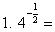2. If a triangle ABC has AB = 3, AC = 4, and BC = 5, then what is the length of the altitude drawn from
vertex A to side BC?

3. If f(x) = 2x3, then f(1+2) =

4. You have some quarters and dimes worth a total of \$7.15. You have three times as many dimes as
quarters. How many coins do you have?

5. The point E is outside the square ABCD so that ABE is an equilateral triangle. What is the number
of degrees in the angle CED?

6. If x, 3x + 1, and 6x + 2 are in geometric progression, then what is x?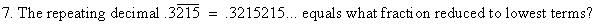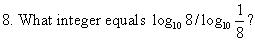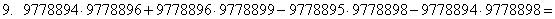10. You start with 128 cents, and six times bet half of your current amount of money, with
probability .5 of winning on each occasion. You win 3 times and lose 3 times in some order.
Does the amount of money which you have at the end depend upon the order of wins and
losses, and if not, how many cents will you have at the end? Your answer should be either the
word "depends" or else the number of cents that you will always have at the end.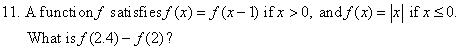12. The number of pairs of positive integers (x,y) which satisfy 2x + 3y = 515 is ?

13. Joe runs around the track at a pace of 80 seconds per lap. Jim runs it in the opposite direction.
They meet every 30 seconds. How many seconds does it take Jim to run each lap around the track?

14. Suppose that the angle between the minute hand and hour hand of a clock is 60 degrees. If the minute
hand is 12 inches long and the hour hand is 9 inches long, then what is the distance in inches between the
tip ends of the hands?

15. If x and y are positive integers less than 100 such that x2 - y2 = 343, then (x,y) =

16. Eight circular coins are arranged so that no two of them overlap or touch, and no three
of them have a tangent line in common. What is the total number of lines that are tangent to
two of the coins?

17. AB is a diameter of a circle of radius 1. If C is any point on the circle, then the possible
values of AC + BC are exactly those numbers s which satisfy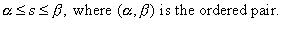18. When 17! is written base 12, how many 0s are at the end?

19. An equaliateral triangle is incribed in a circle of radius 1 with one vertex at the bottom of
the circle. What is the area that lies inside the circle and above the triangle?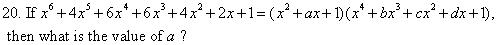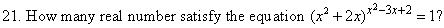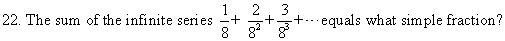23. What positive integer n satisfies log10(225!) - log10(223!)  = l + log10(n!) ?

24. The sum of the first 40 terms of an arithmetic series equals 300, and the sum of the next
40 terms equals 3500. What is the first term of the series?

25. For what real number c does the equation |x2 + 8x + 12| = c have exactly three solutions?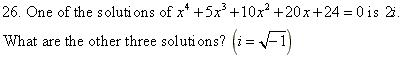27. How many integers between 1000 and 9999 are perfect squares and have sum of
digits equal to 14?

28. For how many paths consisting of a sequence of a horizontal (forward or backward)
and / or vertical line segments, each connecting a pair of adjacent letter in the diagram below,
is the word "COUNT" spelled out as a path is traversed from beginning to end?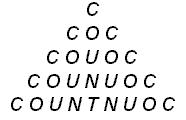29. Mary takes the train home from work each day, arriving at the station at 6:00, at which time
her butler, Jeeves, meets her and drives her home. Jeeves always drives at the same constant
speed, and always arrives at the station at exactly 6:00. One day Mary takes an earlier train
which arrives at the station at 5:00. She immediately begins walking home. On the way, she
meets Jeeves, who is on his way to meet the 6:00train. Mary gets into the car, and they drive
home, arriving 20 minues earlier than usual. How many minutes had Mary been walking?

30. Begin with a circle C with two smaller circles inscribed in it. Step 1: Inscribe a circle into
each non circlular region of the resulting figure. Now repeat Step 1 four more times. How
many of the resulting circles do not touch circle C ? The intital iteration and the first step are
shown below.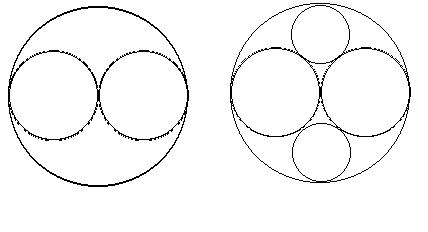31. 25 people are arranged in a circle. Three are selected at random. What is the
probablity that none of those selected were next to one another?

32. How many pairs (m,n) of positive integers have the property that the ratio of the
size of each interior angle of a regular m-gon to that of a regular n-gon equals 3:2?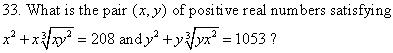34. What is the remainder when  683 + 883 is divided by 49?

35. Let C be the circle of radius 1 centered at (0,0), Give, in simplifed form, a necessary and
sufficient condition on positive numbers x1 and x2 that the line passing through (x2 ,1) and
(x1 ,-1) is tangent to C.

36. The 36th digit after the decimal in the decimal expansion of 1/1999 is

37. Three people are playing a game. They take turns in rotation rolling a fair 6-sided die.
A player who rolls a 5 or 6 wins and the game ends. A player who rollas a 1 or 2  loses and is
out of the game. The game continues until either someone wins (by rolling a 5 or 6) or else there is
only one person left, in which that person wins. What is the probability that the first person to roll
wins?

38. Let 0,A, and B denote the points (0,0,0), (3,0,0) and (0,4,0), respectively. A sphere of
radius 2 in xyz-space is tangent to the lines OA, OB, and AB, and the z-coordinate of its center
is positibe. That are the coordinates of the center of the sphere?

39. How many 4-digit numbers equal (a+b)^2, where a (resp. b) is the number formed by the first (resp. last) two digits of the 4-digit number.

40. Three circles are externally tangent to one another, and all lie inside a circle of radius 4, to
which they are all tangent. Two of the circles have radius 1 and 3. The center of these circles
lie along a diameter of the larger circle. What is the radius of the third circle?